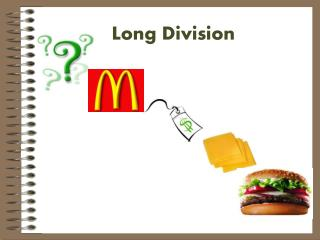DownloadDownload PresentationLong Division

# Long Division

Télécharger la présentation## Long Division

- - - - - - - - - - - - - - - - - - - - - - - - - - - E N D - - - - - - - - - - - - - - - - - - - - - - - - - - -
##### Presentation Transcript

1. Long Division

2. Long Division • Long division is as simple as memorizing this simple question: “Does McDonalds Sell CheeseBurgers?”

3. Each part is a step to completing Long Division! 3. Subtract Sell 1. Divide 4. Check Does Cheese 2. Multiply 5. Bring it Down! McDonalds Burgers

4. First: Terms You Need to Know! 12÷ 4= 3 Dividend Divisor Quotient 3 412

5. Step 1 in Long Division 4 1. Divide • Divide 2 into first number in the dividend. 2 ) 9 4 Does How many 2’s will go into 9? • Think how many 2’s will fit into 9. • Write that number directly above the number you divided into.

6. Step 2 in Long Division 4 × 2. Multiply • Multiply the divisor times the first number in the quotient. 2 ) 9 4 McDonalds 8 2 x 4 = 8 • Write your answer directly under the 9 or the number you just divided into.

7. Step 3 in Long Division 4 3. Subtract • Draw a line under the 8. 2 ) 9 4 Sell 8 • Write a subtraction sign next to the 8. 1 • Subtract 8 from 9. • Write your answer directly below the 8.

8. Step 4 in Long Division 4 2 ) 9 4 Cheese 8 4. Check/Compare to make sure the difference is smaller than the divisor! 1 1 is smaller than 2, so I’m good! • The difference needs to be smaller than • your divisor- or you can divide more!

9. Step 5 in Long Division 4. Bring Down! 4 • Go to the next number in the dividend to the right of the 9. 2 ) 9 4 -Burgers! 8 1 4 • Bring the 4 down next to the 1.

10. REPEAT OR FINISHED? 4 • This is where you decide whether you repeat the 5 steps of division. 2 ) 9 4 8 1 4 • If your divisor can divide into your new number, 14, or if you have numbers in the dividend that have not been brought down, you repeat the 5 steps of division.

11. Step 1 in Long Division 4 7 1. Divide • Divide 2 into your new number, 14. 2 ) 9 4 Does 8 1 4 • Place your answer directly above the 4 in your quotient.

12. Step 2 in Long Division 4 7 2. Multiply × • Multiply your divisor, 2, with your new number in the quotient, 7. 2 ) 9 4 McDonalds 8 1 4 1 4 • Place your answer directly under the 14.

13. Step 3 in Long Division 4 7 3. Subtract • Draw a line under the bottom 14. 2 ) 9 4 Sell 8 1 4 1 4 • Draw a subtraction sign. 0 • Subtract & place answer under the line.

14. Step 4 in Long Division 4 7 2 ) 9 4 Cheese 8 4. Check/Compare to make sure the difference is smaller than the divisor! 1 4 1 4 0 • The difference needs to be • smaller than your divisor- or you • can divide more! 0 is smaller than 2!

15. REPEAT OR FINISHED? 4 7 Quotient • Since there are no more numbers to bring down you do not repeat the 5 steps of division. • The quotient on the top is your answer. • Since you have no numbers left, there is no remainder! 2 ) 9 4 8 1 4 1 4 0 0 Remainder!

16. You’re awesome! 4 7 2 ) 9 4 8 1 4 1 4 You’re so smart! 0 You did it!